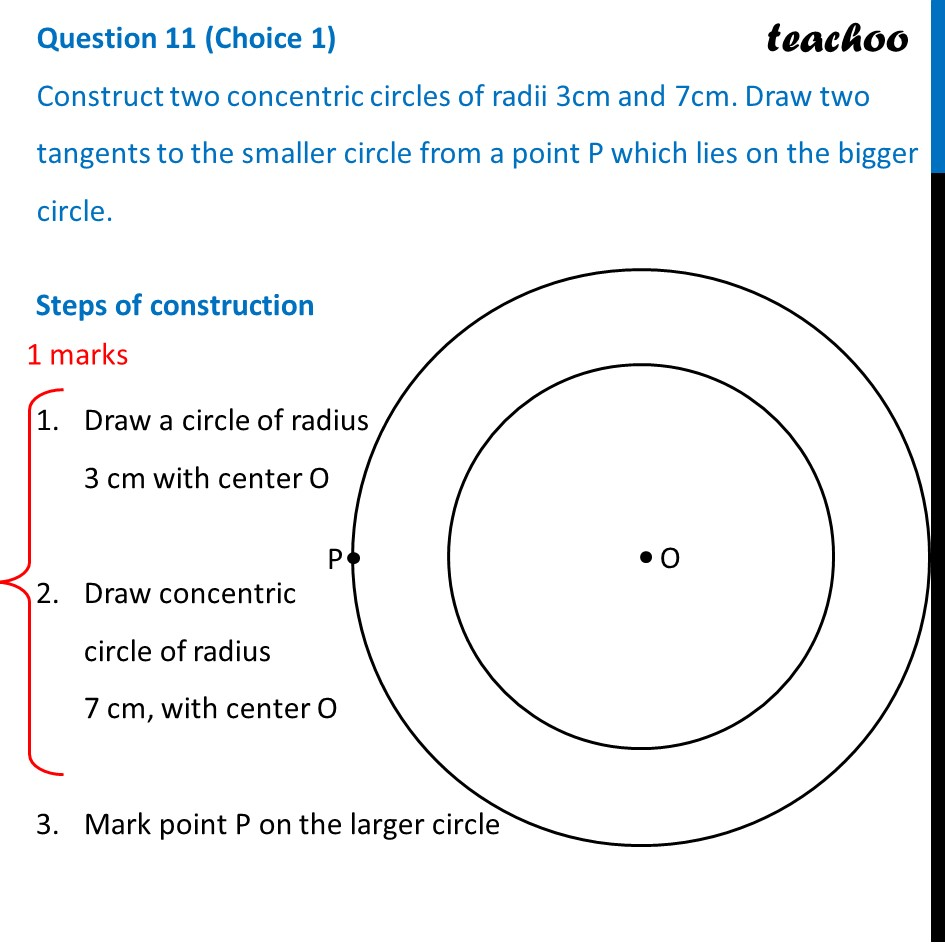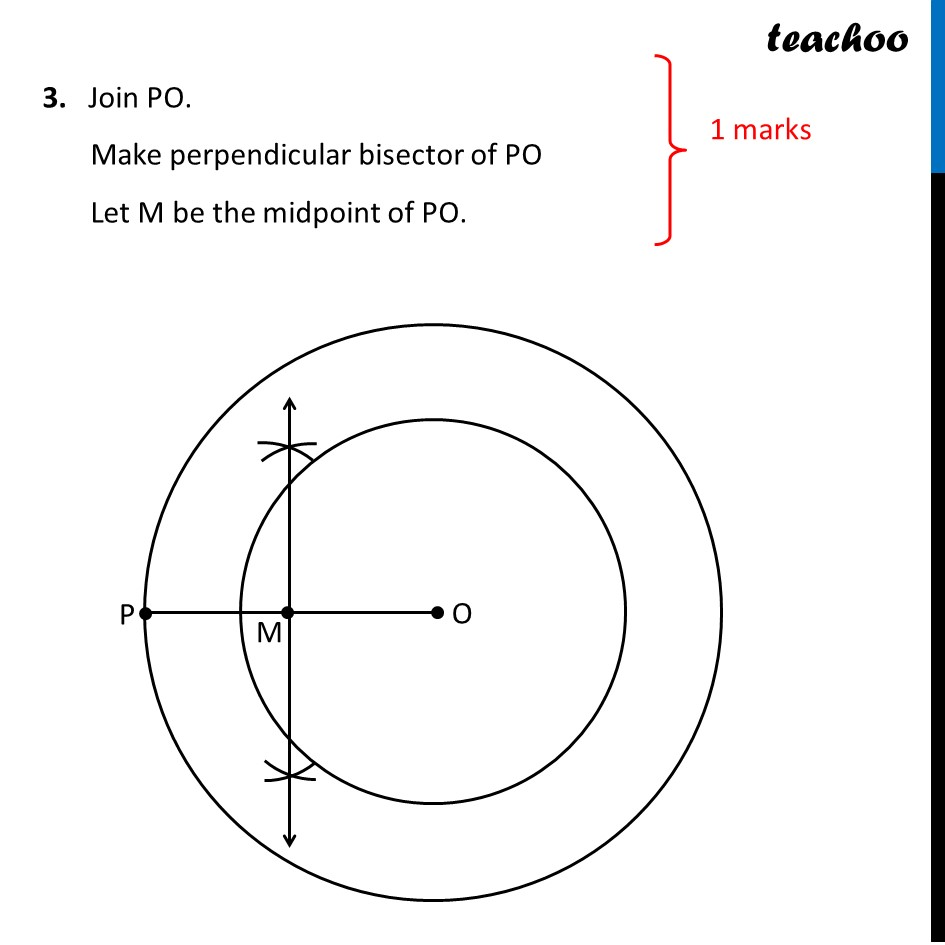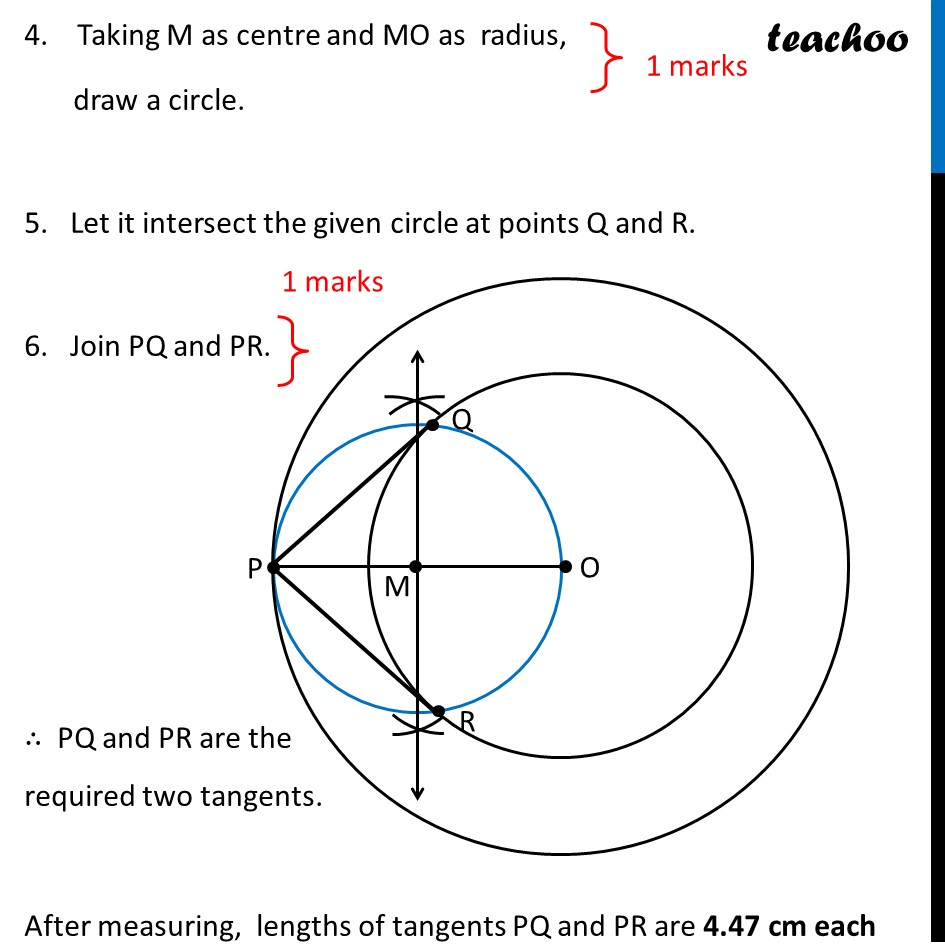CBSE Class 10 Sample Paper for 2022 Boards - Maths Basic [Term 2]

Class 10
Solutions of Sample Papers for Class 10 Boards

## Construct two concentric circles of radii 3cm and 7cm. Draw two tangents to the smaller circle from a point P which lies on the bigger circle.

This question is similar to Ex 11.2, 2 Chapter 11 Class 10 - ConstructionsLearn in your speed, with individual attention - Teachoo Maths 1-on-1 Class

### Transcript

Question 11 (Choice 1) Construct two concentric circles of radii 3cm and 7cm. Draw two tangents to the smaller circle from a point P which lies on the bigger circle. Steps of construction Steps of construction Draw a circle of radius 3 cm with center O Draw concentric circle of radius 7 cm, with center O Mark point P on the larger circle 3. Join PO. Make perpendicular bisector of PO Let M be the midpoint of PO. 4. Taking M as centre and MO as radius, draw a circle. 5. Let it intersect the given circle at points Q and R. 6. Join PQ and PR. ∴ PQ and PR are the required two tangents. After measuring, lengths of tangents PQ and PR are 4.47 cm each